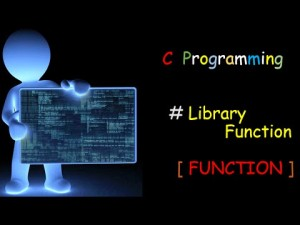10. Why shouldn’t I start variable names with underscores?

Identifier names beginning with two underscores or an underscore followed by a capital letter are reserved for use by the compiler or standard library functions wherever they appear. In addition, all identifier names beginning with an underscore followed by anything are reserved when they appear in file scope (when they are not local to a function).

If you use a reserved identifier for a variable name, the results are undefined (your program might not compile, or it might compile but crash). Even if you are lucky enough to pick an identifier that is not currently used by your compiler or library, remember that these identifiers are reserved for possible use later.

11. What math functions are available for integers? For floating point?

The operations +, -, *, and / (addition, subtraction, multiplication, and division) are available for both integer and floating-point arithmetic. The operator % (remainder) is available for integers only.

For floating-point math, many other functions are declared in the header file math.h. Most of these functions operate in double-precision floating point, for increased accuracy. If these functions are passed an argument outside of their domain (the domain of a function is the set of legal values for which it is defined), the function will return some unspecified value and will set the variable errno to the value EDOM. If the return value of the function is too large or small to be represented by a double (causing overflow or underflow), the function will return HUGE_VAL (for overflow) or 0 (for underflow) and will set errno to ERANGE. The values EDOM, ERANGE, andHUGE_VAL are defined in math.h.

The following list describes the functions declared in math.h:

1. double cos(double), double sin(double), double tan(double) take a value in radians and return the cosine, sine, and tangent of the value, respectively.

2. double acos(double), double asin(double), double atan(double) take a value and return the arc cosine, arc sine, and arc tangent of the value, respectively. The value passed to acos() and asin() must be in the range -1 to 1, inclusive.

3. double atan2(double x, double y) returns the arc tangent of the value represented by x/y, even if x/yis not representable as a double (if y is 0, for instance).

4. double cosh(double), double sinh(double), double tanh(double) take a value in radians and return the hyperbolic cosine, hyperbolic sine, and hyperbolic tangent of the value, respectively.

5. double exp(double x), double log(double x), double log10(double x) take a value and return ex, the natural logarithm of x, and the logarithm base 10 of x, respectively. The two logarithm functions will cause a range error (ERANGE) if x is 0 and a domain error (EDOM) if x is negative.

6. double sqrt(double) returns the square root of its argument. It causes a domain error (EDOM) if the value passed to it is negative.

7. double ldexp(double n, int e) returns n * 2e. This is somewhat analogous to the << operator for integers.

8. double pow(double b, double e) returns be. It causes a domain error (EDOM) if b is 0 and e is less than or equal to 0, or if b is less than 0 and e is not an integral value.

9. double frexp(double n, int *i) returns the mantissa of n and sets the int pointed to by i to the exponent of n. The mantissa is in the range 0.5 to 1 (excluding 1 itself ), and the exponent is a number such that n = mantissa * 2exponent.

10. double modf(double n, int *i) returns the fractional part of n and sets the int pointed to by i to the integer part of n.